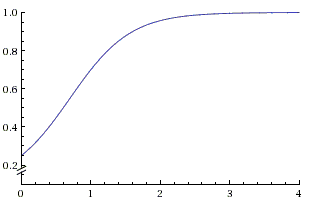# Conditional Recurrence

When tossing a coin repeatedly, the probability of any outcome on any particular event is independent of the preceding outcomes. Assuming the coin is fair and shows either heads or tails with the probability $\frac{1}{2}$, the probability remains the same on the one thousands even in the unlikely event that all the previous outcomes were all, say, heads. The independences of the events in this case means that, for example,

$P(HH|H) = \frac{1}{2}$,

meaning that the conditional probability of getting heads ($H$) on the second throw remains the same even if heads came up on the first. If the coin is not necessarily fair and shows heads with the probability of $p$, still

$P(HH|H) = p$, and $P(HHH|HH) = p$, etc.

There is a situation bewildering to many when that is not the case. More accurately, there are circumstances, where the past events affect the probability of the subsequent ones, even though the individual experiments are independent.

Let there be two coins that come up heads with the probabilities $p$ and $q$, $p \gt q$. At the outset, we choose one of the coins randomly, say, we choose the first coin with the probability $r$ and the other with the probability $1 - r$. Then, the probability of having $k$ heads $(H^k)$ in a row is given by

$P(H^k) = rp^k + (1-r)q^k$.

This allows us to compute the conditional probability $P(H^{k+1}|H^k)$:

$\displaystyle P(H^{k+1}|H^k) = \frac{P(H^{k+1})}{P(H^k)} = \frac{rp^{k+1} + (1-r)q^{k+1}}{rp^k + (1-r)q^k} = p\frac{r + (1-r)(q/p)^{k+1}}{r + (1-r)(q/p)^{k}}$.

Since $p \gt q$, both $r + (1-r)(q/p)^{k+1}$ and $r + (1-r)(q/p)^{k}$ tend to $r$ as $k\rightarrow\infty$, so that as the number of experiments $k$ grows, $P(H^{k+1}|H^k)$ approaches $p$. But it attains the value of $p$ for a finite number of experiments.

Taking an example from the classic [Feller], let $p = 0.6$, $q = 0.06$, and $\displaystyle r = \frac{1}{6}$. Then $P(H) = 0.15$, $P(HH) = 0.063$, so that $\displaystyle P(HH|H) = \frac{0.063}{0.15} = 0.42$, which is rather different from $0.15$. $P(HHH) = 0.03618$, while $P(HHH|HH)\approx 0.574$.

Here's the graph of function $\displaystyle f(t) = \frac{1+5\cdot .1^{t+1}}{1+5\cdot .1^t}$ produced by wolframalphaFeller mentions that this reasoning is employed by insurance companies to estimate the premium for clients who are prone to accidents or a particular kind of illness. A naturalist who came across a rare insect may expect to discover a few more of the specimen for insects live in colonies. A similar reasoning works for a mushroom hunter, since mushrooms seldom grow in solitude.

### References

1. W. Feller, An Introduction to Probability Theory and Its Applications, Vol. 1, Wiley, 3rd Edition, 1968, V.2